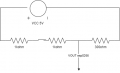# Wrong signal from a voltage divider wit esp8266

Joined Aug 28, 2022
8
I have the following circuit.I connected vout with A0 pin(adc pin) of my esp8266. When I read the tension by a0(with command analogRead(A0)), I expect that value is about 667(0.652 V). But instead I get a random signal(not const)... by analogRead(A0) i get:
etc...

I don't understand why...
PS: As a power supply I use a bench power supply and I already try to change esp8266 with another(to try if it if broken...)

#### Ian0

Joined Aug 7, 2020
8,378
To ask the obvious question . . .
is the negative terminal of your supply (in the diagram) connected to the Vss of the ESP8266?

Joined Aug 28, 2022
8
Try connecting the 3.3V of the ESP to A0 input, you should read 1023.
Then try with A0 at 0V, should get 0000

E

BTW: I assume you have connected the 0V of the power supply to the 0V of the ESP.????

View attachment 276729
No, I did not it. At the esp I only connected the a0 pin and the usb for the power supply. For you, do i need to connect it to ground of esp?

#### ericgibbs

Joined Jan 29, 2010
17,980
For you, do i need to connect it to ground of esp?
Yes.
E

Joined Aug 28, 2022
8
Yes.
E
Now works!!
Vout = vin *(r2 / (r1 + r2)) = 5 * 300 / (2000 + 300) = 0.65v

#### ericgibbs

Joined Jan 29, 2010
17,980
hi d,
Is the ESP a 12 Bit ADC ie: 4095.??? for 5V

E

#### Ian0

Joined Aug 7, 2020
8,378
I guess that the positive reference for the ADC is connected to 5V.
So that the reading would be
Vin/5V * 1024

Joined Jan 15, 2015
7,217
The ESP8266 is a 10 bit ADC and uses a 3.3 volt V reference. Also the ESP8266 is non linear when measuring voltage especially levels below 1.0 volt. You can oversample, take many samples and average but keep in mind you can't make a silk purse from a sows ear. Some chips respond better than others.

To convert to reading voltage the code would look like this or similar:
Code:
const int analogInPin = A0;  // ESP8266 Analog Pin ADC0 = A0

int sensorValue = 0;  // value read from the pot
float volts;

void setup() {
// initialize serial communication at 115200
Serial.begin(115200);
}

void loop() {

volts = (sensorValue * 3.3 / 1024);
// print the readings in the Serial Monitor
Serial.print("sensor = ");
Serial.println(sensorValue);

Serial.print ("Volts =  ");
Serial.println (volts);

delay(1000);
}
The result would look like this with 0.652 volts applied.Note the standard voltage applied is 0.652 volts. You can see the low end error. Using your divider your output would be close to the above input.

Ron

Ron

Last edited: